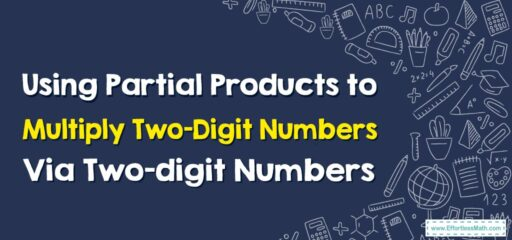# How to Use Partial Products to Multiply Two-Digit Numbers By Two-digit Numbers

Using the Partial Products method to multiply two-digit numbers helps you visualize the multiplication process.## A Step-by-step Guide to Using Partial Products to Multiply Two-Digit Numbers By Two-digit Numbers

Here’s a step-by-step guide to using the Partial Products method to multiply two-digit numbers. We’ll use the problem $$24×35$$ as an example.

### Step 1: Break down the numbers into tens and ones

The first step is to break down both numbers into their tens and ones:

• 24 is broken down into $$20+4$$
• 35 is broken down into $$30+5$$

### Step 2: Multiply each part of the first number with each part of the second number

Now, you multiply each part of the first number with each part of the second number:

• 20 (from 24) $$\times30$$ (from 35) = 600
• 20 (from 24) $$\times5$$ (from 35) = 100
• 4 (from 24) $$\times30$$ (from 35) = 120
• 4 (from 24) $$\times5$$ (from 35) = 20

### Step 3: Add up all the products

$$600+100+120+20=840$$
So, $$24\times35=840$$

This method is called the Partial Products method because you are finding the product of parts of the numbers (the “partial products”) and then adding them together. This method helps students understand the concept of multiplication and the importance of place value. It can be a great stepping stone before learning more traditional multiplication algorithms.

### What people say about "How to Use Partial Products to Multiply Two-Digit Numbers By Two-digit Numbers - Effortless Math: We Help Students Learn to LOVE Mathematics"?

No one replied yet.

X
30% OFF

Limited time only!

Save Over 30%

SAVE $5 It was$16.99 now it is \$11.99